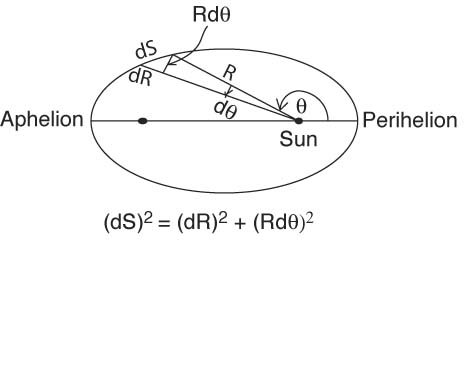Orbits of Planets are EllipsesAssume a planet with mass M orbiting the Sun with mass S.  Conservation of energy can be written as

(1) P.E. + K.E. = E

1/2 Mv2  - GMS/R = E

The velocity v along the orbit is the derivative dS/dt.  Hence,

v2 = (dS/dt)2 , which can be written as

(2) v2 = (dS/dt)2 = (dR/dt)2 + R2(dq/dt)2

by noticing from the diagram above that (dS)2 = (dR)2 + R2(dq).

Using conservation of angular momentum L = I w = MR2 dq/dt = constant, we can substitute dq/dt = L/MR2 in equation (2) for v2.  Then equation (1) for conservation of energy becomes

E = 1/2 (L2/(MR4)) [ (dR/dq)2 + R2 ]  - G (MS/R)

Rearranging terms gives

[ (dR/dq)2 + R2 ] = (2EMR4/L2)  + (2GSM2R3/L2)

The derivative dR/dq  thus can be written as

(3) dR/dq = R[(2EM/L2) R2 + 2 GSM2/L2 R - 1 ] 1/2

defining combinations of constants p = L2/GSM2 and (e2 - 1)= 2EL2/(G2S2M3)  equation (3) can be written as

(4) dR/dq = R [(e2 - 1)/p2 R2   +  (2/p) R - 1 ] 1/2

# The differential equation (4) above has a solution that can be verified by differentiation:

(5) R = p /(1 + e cos (q + c))

This is the equation for an ellipse with the origin at one focus (the sun).  The constant of integration c is zero if q = 0 for Rp at perihelion and q = p for Ra at aphelion.

Rp = p/(1+e)

Ra = p/(1-e)

Since the semi-major axis of the ellipse is 2a = Rp + Ra, we have

p = a (1- e2)

Consider four different situations for the ratio of the magnitude of potential energy to kinetic energy and what they imply for the eccentricity e

(1)         P.E. > K.E.

The total E is negative and since

e2 = 2EL2/(G2S2M3) + 1 , e2 < 1 and the path of the planet is an ellipse with semimajor axis a and semiminor axis c = a(1- e2)1/2

(2)         P.E. = K.E.

When E = 0, the eccentricity  e is zero, describing a parabola.  This is the condition for escape.

(3)         P.E. < K.E.

E > 0 , the eccentricity  e is greater than 1 and the path is hyperbolic

(4)         When eccentricity e is zero:

e = 2EL2/(G2S2M3) + 1 = 0

or E = - G2S2M3/2L2

This is the minimum value (largest negative value) that E (total energy) can take for any orbital path.   The corresponding K.E. is the minimum value of K.E. for any possible conic section. The orbit is a circle.